# On the structural law of exoplanetary systems.pptx

29. May 2023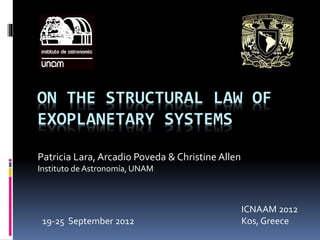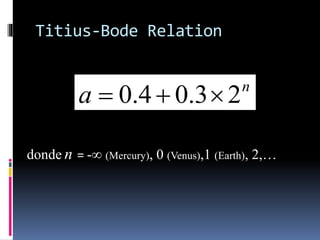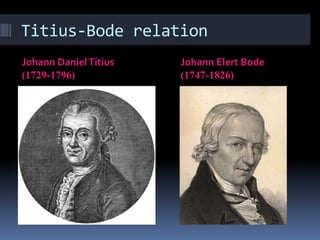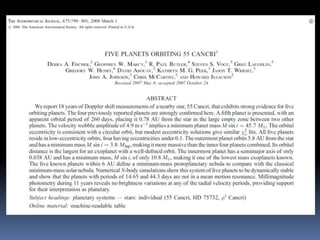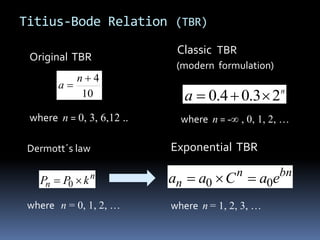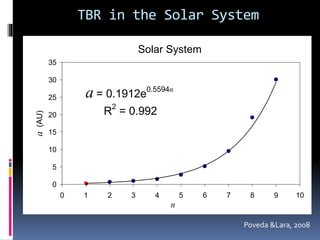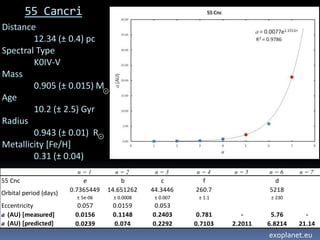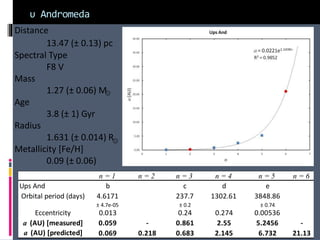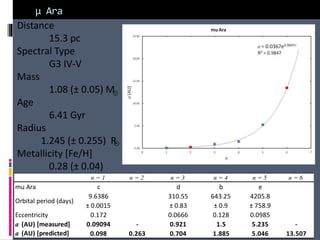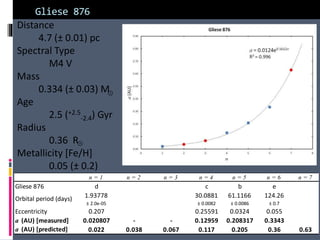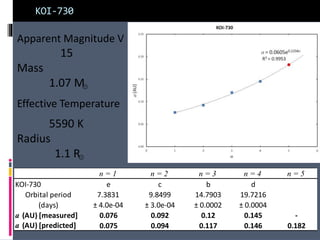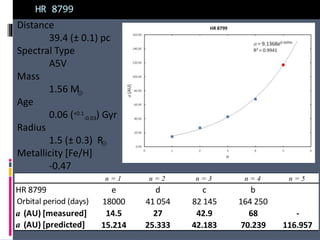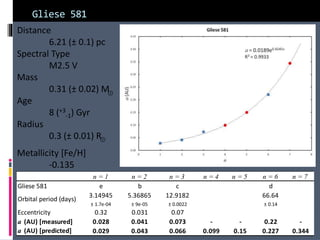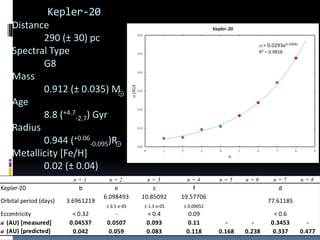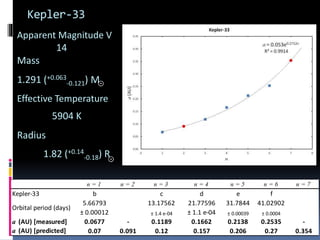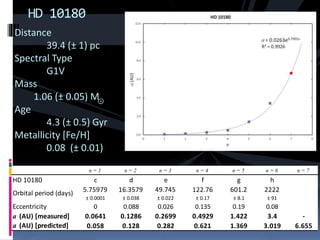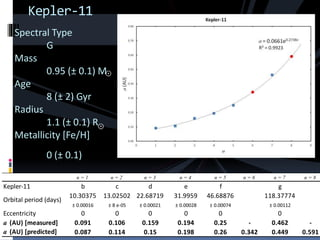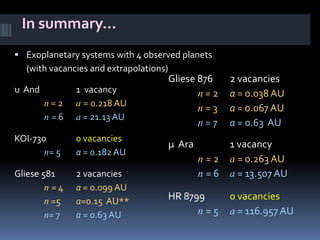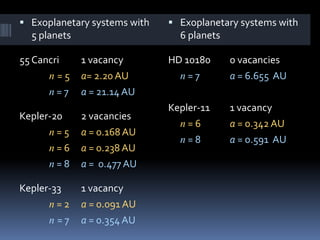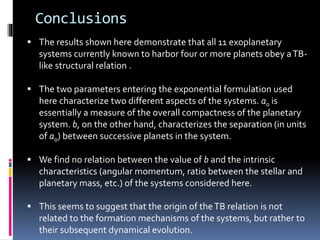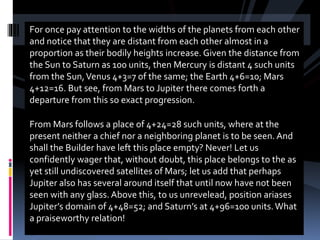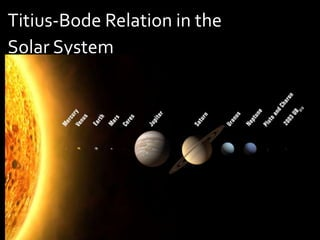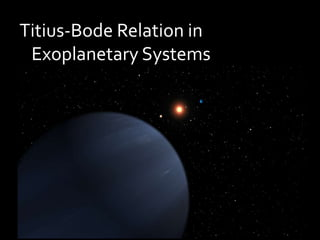1 von 25

### On the structural law of exoplanetary systems.pptx

• 1. ON THE STRUCTURAL LAW OF EXOPLANETARY SYSTEMS Patricia Lara, Arcadio Poveda & Christine Allen Instituto de Astronomía, UNAM ICNAAM 2012 Kos, Greece 19-25 September 2012
• 2. Titius-Bode Relation n a 2 3 . 0 4 . 0    donde n = -∞ (Mercury), 0 (Venus),1 (Earth), 2,…
• 5. Titius-Bode Relation (TBR) Original TBR where n = 0, 3, 6,12 .. Classic TBR (modern formulation) where n = -∞ , 0, 1, 2, … 10 4   n a n a 2 3 . 0 4 . 0    Exponential TBR where n = 1, 2, 3, … n n k P P   0 Dermott´s law where n = 0, 1, 2, … bn n n e a C a a 0 0   
• 6. TBR in the Solar System Sistema Solar a = 0.1912e0.5594n R 2 = 0.992 0 5 10 15 20 25 30 35 0 1 2 3 4 5 6 7 8 9 10 n a (AU) Poveda &Lara, 2008 Solar System
• 8. 55 Cancri Distance 12.34 (± 0.4) pc Spectral Type K0IV-V Mass 0.905 (± 0.015) M Age 10.2 (± 2.5) Gyr Radius 0.943 (± 0.01) R Metallicity [Fe/H] 0.31 (± 0.04) n = 1 n = 2 n = 3 n = 4 n = 5 n = 6 n = 7 55 Cnc e b c f d Orbital period (days) 0.7365449 ± 5e-06 14.651262 ± 0.0008 44.3446 ± 0.007 260.7 ± 1.1 5218 ± 230 Eccentricity 0.057 0.0159 0.053 a (AU) [measured] 0.0156 0.1148 0.2403 0.781 - 5.76 - a (AU) [predicted] 0.0239 0.074 0.2292 0.7103 2.2011 6.8214 21.14 exoplanet.eu
• 9. υ Andromeda Distance 13.47 (± 0.13) pc Spectral Type F8 V Mass 1.27 (± 0.06) M Age 3.8 (± 1) Gyr Radius 1.631 (± 0.014) R Metallicity [Fe/H] 0.09 (± 0.06) n = 1 n = 2 n = 3 n = 4 n = 5 n = 6 Ups And b c d e Orbital period (days) 4.6171 ± 4.7e-05 237.7 ± 0.2 1302.61 3848.86 ± 0.74 Eccentricity 0.013 0.24 0.274 0.00536 a (AU) [measured] 0.059 - 0.861 2.55 5.2456 - a (AU) [predicted] 0.069 0.218 0.683 2.145 6.732 21.13
• 10. μ Ara Distance 15.3 pc Spectral Type G3 IV-V Mass 1.08 (± 0.05) M Age 6.41 Gyr Radius 1.245 (± 0.255) R Metallicity [Fe/H] 0.28 (± 0.04) n = 1 n = 2 n = 3 n = 4 n = 5 n = 6 mu Ara c d b e Orbital period (days) 9.6386 ± 0.0015 310.55 ± 0.83 643.25 ± 0.9 4205.8 ± 758.9 Eccentricity 0.172 0.0666 0.128 0.0985 a (AU) [measured] 0.09094 - 0.921 1.5 5.235 - a (AU) [predicted] 0.098 0.263 0.704 1.885 5.046 13.507
• 11. Gliese 876 Distance 4.7 (± 0.01) pc Spectral Type M4 V Mass 0.334 (± 0.03) M Age 2.5 (+2.5 -2.4) Gyr Radius 0.36 R Metallicity [Fe/H] 0.05 (± 0.2) n = 1 n = 2 n = 3 n = 4 n = 5 n = 6 n = 7 Gliese 876 d c b e Orbital period (days) 1.93778 ± 2.0e-05 30.0881 ± 0.0082 61.1166 ± 0.0086 124.26 ± 0.7 Eccentricity 0.207 0.25591 0.0324 0.055 a (AU) [measured] 0.020807 - - 0.12959 0.208317 0.3343 a (AU) [predicted] 0.022 0.038 0.067 0.117 0.205 0.36 0.63
• 12. KOI-730 Apparent Magnitude V 15 Mass 1.07 M Effective Temperature 5590 K Radius 1.1 R n = 1 n = 2 n = 3 n = 4 n = 5 KOI-730 e c b d Orbital period (days) 7.3831 ± 4.0e-04 9.8499 ± 3.0e-04 14.7903 ± 0.0002 19.7216 ± 0.0004 a (AU) [measured] 0.076 0.092 0.12 0.145 - a (AU) [predicted] 0.075 0.094 0.117 0.146 0.182
• 13. HR 8799 Distance 39.4 (± 0.1) pc Spectral Type A5V Mass 1.56 M Age 0.06 (+0.1 -0.03) Gyr Radius 1.5 (± 0.3) R Metallicity [Fe/H] -0.47 n = 1 n = 2 n = 3 n = 4 n = 5 HR 8799 e d c b Orbital period (days) 18000 41 054 82 145 164 250 a (AU) [measured] 14.5 27 42.9 68 - a (AU) [predicted] 15.214 25.333 42.183 70.239 116.957
• 14. Gliese 581 Distance 6.21 (± 0.1) pc Spectral Type M2.5 V Mass 0.31 (± 0.02) M Age 8 (+3 -1) Gyr Radius 0.3 (± 0.01) R Metallicity [Fe/H] -0.135 n = 1 n = 2 n = 3 n = 4 n = 5 n = 6 n = 7 Gliese 581 e b c d Orbital period (days) 3.14945 ± 1.7e-04 5.36865 ± 9e-05 12.9182 ± 0.0022 66.64 ± 0.14 Eccentricity 0.32 0.031 0.07 a (AU) [measured] 0.028 0.041 0.073 - - 0.22 - a (AU) [predicted] 0.029 0.043 0.066 0.099 0.15 0.227 0.344
• 15. Kepler-20 Distance 290 (± 30) pc Spectral Type G8 Mass 0.912 (± 0.035) M Age 8.8 (+4.7 -2.7) Gyr Radius 0.944 (+0.06 -0.095)R Metallicity [Fe/H] 0.02 (± 0.04) n = 1 n = 2 n = 3 n = 4 n = 5 n = 6 n = 7 n = 8 Kepler-20 b e c f d Orbital period (days) 3.6961219 6.098493 ± 6.5 e-05 10.85092 ± 1.3 e-05 19.57706 ± 0.00052 77.61185 Eccentricity < 0.32 - < 0.4 0.09 < 0.6 a (AU) [measured] 0.04537 0.0507 0.093 0.11 - - 0.3453 - a (AU) [predicted] 0.042 0.059 0.083 0.118 0.168 0.238 0.337 0.477
• 16. Kepler-33 n = 1 n = 2 n = 3 n = 4 n = 5 n = 6 n = 7 Kepler-33 b c d e f Orbital period (days) 5.66793 ± 0.00012 13.17562 ± 1.4 e-04 21.77596 ± 1.1 e-04 31.7844 ± 0.00039 41.02902 ± 0.0004 a (AU) [measured] 0.0677 - 0.1189 0.1662 0.2138 0.2535 - a (AU) [predicted] 0.07 0.091 0.12 0.157 0.206 0.27 0.354 Apparent Magnitude V 14 Mass 1.291 (+0.063 -0.121) M Effective Temperature 5904 K Radius 1.82 (+0.14 -0.18) R
• 17. HD 10180 Distance 39.4 (± 1) pc Spectral Type G1V Mass 1.06 (± 0.05) M Age 4.3 (± 0.5) Gyr Metallicity [Fe/H] 0.08 (± 0.01) n = 1 n = 2 n = 3 n = 4 n = 5 n = 6 n = 7 HD 10180 c d e f g h Orbital period (days) 5.75979 ± 0.0001 16.3579 ± 0.038 49.745 ± 0.022 122.76 ± 0.17 601.2 ± 8.1 2222 ± 91 Eccentricity 0 0.088 0.026 0.135 0.19 0.08 a (AU) [measured] 0.0641 0.1286 0.2699 0.4929 1.422 3.4 - a (AU) [predicted] 0.058 0.128 0.282 0.621 1.369 3.019 6.655
• 18. Kepler-11 Spectral Type G Mass 0.95 (± 0.1) M Age 8 (± 2) Gyr Radius 1.1 (± 0.1) R Metallicity [Fe/H] 0 (± 0.1) n = 1 n = 2 n = 3 n = 4 n = 5 n = 6 n = 7 n = 8 Kepler-11 b c d e f g Orbital period (days) 10.30375 ± 0.00016 13.02502 ± 8 e-05 22.68719 ± 0.00021 31.9959 ± 0.00028 46.68876 ± 0.00074 118.37774 ± 0.00112 Eccentricity 0 0 0 0 0 0 a (AU) [measured] 0.091 0.106 0.159 0.194 0.25 - 0.462 - a (AU) [predicted] 0.087 0.114 0.15 0.198 0.26 0.342 0.449 0.591
• 19. In summary…  Exoplanetary systems with 4 observed planets (with vacancies and extrapolations) υ And 1 vacancy n = 2 a = 0.218 AU n = 6 a = 21.13 AU KOI-730 0 vacancies n= 5 a = 0.182 AU Gliese 581 2 vacancies n = 4 a = 0.099 AU n =5 a=0.15 AU** n= 7 a = 0.63 AU Gliese 876 2 vacancies n = 2 a = 0.038 AU n = 3 a = 0.067 AU n = 7 a = 0.63 AU μ Ara 1 vacancy n = 2 a = 0.263 AU n = 6 a = 13.507 AU HR 8799 0 vacancies n = 5 a = 116.957 AU
• 20.  Exoplanetary systems with 5 planets 55 Cancri 1 vacancy n = 5 a= 2.20 AU n = 7 a = 21.14 AU Kepler-20 2 vacancies n = 5 a = 0.168 AU n = 6 a = 0.238 AU n = 8 a = 0.477 AU Kepler-33 1 vacancy n = 2 a = 0.091 AU n = 7 a = 0.354 AU  Exoplanetary systems with 6 planets HD 10180 0 vacancies n = 7 a = 6.655 AU Kepler-11 1 vacancy n = 6 a = 0.342 AU n = 8 a = 0.591 AU
• 21. Conclusions  The results shown here demonstrate that all 11 exoplanetary systems currently known to harbor four or more planets obey aTB- like structural relation .  The two parameters entering the exponential formulation used here characterize two different aspects of the systems. a0 is essentially a measure of the overall compactness of the planetary system. b, on the other hand, characterizes the separation (in units of a0) between successive planets in the system.  We find no relation between the value of b and the intrinsic characteristics (angular momentum, ratio between the stellar and planetary mass, etc.) of the systems considered here.  This seems to suggest that the origin of theTB relation is not related to the formation mechanisms of the systems, but rather to their subsequent dynamical evolution.
• 23. For once pay attention to the widths of the planets from each other and notice that they are distant from each other almost in a proportion as their bodily heights increase. Given the distance from the Sun to Saturn as 100 units, then Mercury is distant 4 such units from the Sun,Venus 4+3=7 of the same; the Earth 4+6=10; Mars 4+12=16. But see, from Mars to Jupiter there comes forth a departure from this so exact progression. From Mars follows a place of 4+24=28 such units, where at the present neither a chief nor a neighboring planet is to be seen. And shall the Builder have left this place empty? Never! Let us confidently wager that, without doubt, this place belongs to the as yet still undiscovered satellites of Mars; let us add that perhaps Jupiter also has several around itself that until now have not been seen with any glass. Above this, to us unrevelead, position ariases Jupiter’s domain of 4+48=52; and Saturn’s at 4+96=100 units.What a praiseworthy relation!
• 24. Titius-Bode Relation in the Solar System

### Hinweis der Redaktion

1. First I would like thank yo the organizing comitte for invite me to this event I´m here to present my work that I being doing with my advisors Dr. Arcadio Poveda and Mrs Christine Allen The title of this talk is On the structural law fo exoplanetary systems Well What is it about?
2. The major semi-axes of the Solar System obey a simple geometric progression know the Titius-Bode Law. The Titius-Bode Law is not really a law in the physical sense but rather a numerical relation. SO because of that we will call it Titius-Bode relation or TBR. Here is the mathematical relation for the Solar system where “a” gives the distance in astronomical units for the corresponding orbital number. The TBR was born and prevale until our days because of two astronomers: Johann Daniel Titius and Johann Elert Bode Titius was the first to declare it and Bode using his fame and prestige promote it every time that he has oportunity.
3. The discovery of the fith planet in 55 cancri (THAT AT THE TIME WAS THE EXOPLANETARY SYSTEM WITH MOST PLANETS OBSERVED) Who motivated us to try TBR to this exoplanetary system. Here we will show that the others 10 systems to harbor four or more planets also obey a structural law.
4. If we put in a mathematical form what Titius state orally, the ecuation will be like this… We can see that Titius scaleated the distance from the Sun to the Earth to 10 units so the orbital number follow this progression where for Mercury is 0, 3 for Venus, 6 to the Earth. Through the years the Original TBR suffer modifications for the Solar System in the orbital number, here we can observed that for Mercury correspond –infinity, 0 for Venus, 1 for the Earth and so on. In 1968, Dermott et al shown that the major satellite of Jupiter, Saturn and Uranus obey a similar progression of… For Keplerian orbits Dermorr’s law will obey a similar law like this….. And the two parameters to stablish in this exponential relation is Ao and B.
5. For the porpuose that TBR could be a structural law for planetary systems, it has to be a generalized relation. So we applied to the solar system. Here I show the fit to the Solar system where in the horizontal axis is the orbital number and in the vertical axis is the distances in astronomic units, and looks very well and this fit along with 55 cancri was published in paper of Poveda and Lara, for 55 Cancri we will see later his fit
6. Also we apply TBR to the major satellite of Jupiter, Saturn and Uranus. And every fit looks good and here we have to pay attention to the Gallilean Satellite. Io, Europe and Ganimedes are in resonance know as the Laplacian resonance (1:2:4) and this system is an exemplar example of the TBR.
7. This the fit for 55 Cancri, and here we observed the orbital parameters and the observed distances. In the paper of Poveda and Lara, 55 Cancri e has a major semi-axes of 0.038 but in 2010 Dawson et al argued that the major semi-axis of 55 Cancri is 0.0156. Because of that I show the fit with the corrected distance. This fit show a vacancy in the orbital 5, and we predict a planet as yet to be found at 2.2 AU. Also we extrapolate to the orbital 7 and with less ceartanty we predict a planet in the orbital 7 with a major semi-axis of 21.14
8. Now we start to analize the exoplanetary systems with 4 planets. We see the the exoplanetary system of Upsilon Andromeda. Also we the observed and predicted distances by TBR. TBR fir show a vacancy in the orbital 2 with a major semi-axis of